Next: 4-velocity and 4-acceleration Up: Relativity and electromagnetism Previous: Space-time

## Proper time

It is often helpful to write the invariant differential intervalin the form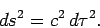(1419)

The quantity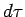is called the proper time. It follows that(1420)

Consider a series of events on the world-line of some material particle. If the particle has speed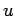then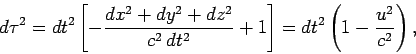(1421)

implying that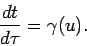(1422)

It is clear thatin the particle's rest frame. Thus,corresponds to the time difference between two neighbouring events on the particle's world-line, as measured by a clock attached to the particle (hence, the name proper time). According to Eq. (1422), the particle's clock appears to run slow, by a factor, in an inertial frame in which the particle is moving with velocity. This is the celebrated time dilation effect.

Let us consider how a small 4-dimensional volume element in space-time transforms under a general Lorentz transformation. We have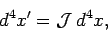(1423)

where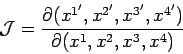(1424)

is the Jacobian of the transformation: i.e., the determinant of the transformation matrix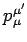. A general Lorentz transformation is made up of a standard Lorentz transformation plus a displacement and a rotation. Thus, the transformation matrix is the product of that for a standard Lorentz transformation, a translation, and a rotation. It follows that the Jacobian of a general Lorentz transformation is the product of that for a standard Lorentz transformation, a translation, and a rotation. It is well-known that the Jacobian of the latter two transformations is unity, since they are both volume preserving transformations which do not affect time. Likewise, it is easily seen [e.g., by taking the determinant of the transformation matrix (1401)] that the Jacobian of a standard Lorentz transformation is also unity. It follows that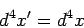(1425)

for a general Lorentz transformation. In other words, a general Lorentz transformation preserves the volume of space-time. Since time is dilated by a factor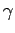in a moving frame, the volume of space-time can only be preserved if the volume of ordinary 3-space is reduced by the same factor. As is well-known, this is achieved by length contraction along the direction of motion by a factor.Next: 4-velocity and 4-acceleration Up: Relativity and electromagnetism Previous: Space-time
Richard Fitzpatrick 2006-02-02